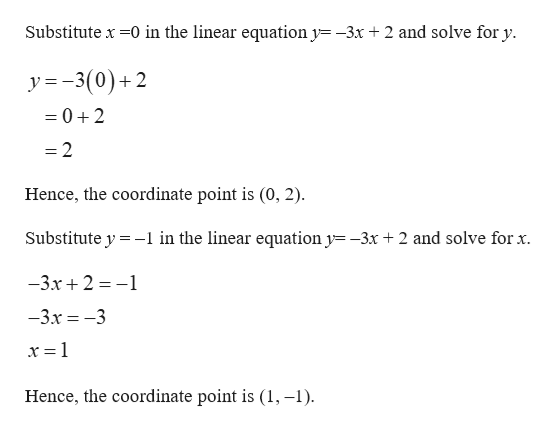# How do you graph y= -3x + 2

Question

How do you graph y= -3x + 2

check_circleExpert Solution
Step 1

Consider the given equation as y= –3x + 2.

Since the equation is linear, its graph will be straight line with slope –3 and the y intercept is 2.

In order to plot a ...help_outlineImage TranscriptioncloseSubstitute x 0 in the linear equation y -3x + 2 and solve for y y = -3(0)+2 =0+2 =2 Hence, the coordinate point is (0, 2) Substitute y -1 in the linear equation y= -3x +2 and solve for x. -3.x2-1 -3.x-3 x = 1 Hence, the coordinate point is (1, -1) fullscreen

### Want to see the full answer?

See Solution

#### Want to see this answer and more?

Solutions are written by subject experts who are available 24/7. Questions are typically answered within 1 hour*

See Solution
*Response times may vary by subject and question
Tagged in

### Other